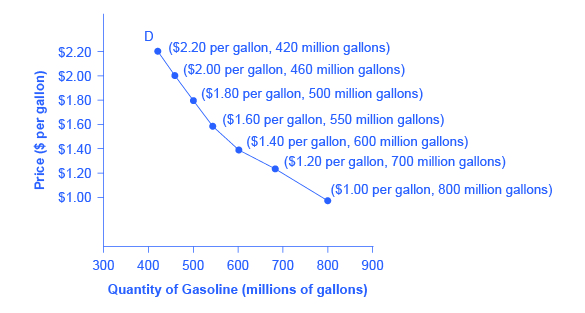# Demand, Supply, and Equilibrium in Markets for Goods and Services

## Demand for Goods and Services

Economists use the term demand to refer to the amount of some good or service consumers are willing and able to purchase at each price. Demand is fundamentally based on needs and wants—if you have no need or want for something, you won't buy it. While a consumer may be able to differentiate between a need and a want, but from an economist’s perspective they are the same thing. Demand is also based on ability to pay. If you cannot pay for it, you have no effective demand. By this definition, a homeless person probably has no effective demand for shelter.

What a buyer pays for a unit of the specific good or service is called price. The total number of units that consumers would purchase at that price is called the quantity demanded. A rise in price of a good or service almost always decreases the quantity demanded of that good or service. Conversely, a fall in price will increase the quantity demanded. When the price of a gallon of gasoline increases, for example, people look for ways to reduce their consumption by combining several errands, commuting by carpool or mass transit, or taking weekend or vacation trips closer to home. Economists call this inverse relationship between price and quantity demanded the law of demand. The law of demand assumes that all other variables that affect demand (which we explain in the next module) are held constant.

We can show an example from the market for gasoline in a table or a graph. Economist call a table that shows the quantity demanded at each price, such as Table, a demand schedule. In this case we measure price in dollars per gallon of gasoline. We measure the quantity demanded in millions of gallons over some time period (for example, per day or per year) and over some geographic area (like a state or a country). A demand curve shows the relationship between price and quantity demanded on a graph like Figure, with quantity on the horizontal axis and the price per gallon on the vertical axis. (Note that this is an exception to the normal rule in mathematics that the independent variable (x) goes on the horizontal axis and the dependent variable (y) goes on the vertical. Economics is not math.)

Table shows the demand schedule and the graph in Figure shows the demand curve. These are two ways to describe the same relationship between price and quantity demanded.

Price (per gallon) Quantity Demanded (millions of gallons)
$1.00 800$1.20 700
$1.40 600$1.60 550
$1.80 500$2.00 460
\$2.20 420
Price and Quantity Demanded of GasolineA Demand Curve for Gasoline The demand schedule shows that as price rises, quantity demanded decreases, and vice versa. We graph these points, and the line connecting them is the demand curve (D). The downward slope of the demand curve again illustrates the law of demand—the inverse relationship between prices and quantity demanded.

Demand curves will appear somewhat different for each product. They may appear relatively steep or flat, or they may be straight or curved. Nearly all demand curves share the fundamental similarity that they slope down from left to right. Demand curves embody the law of demand: As the price increases, the quantity demanded decreases, and conversely, as the price decreases, the quantity demanded increases.

Confused about these different types of demand? Read the next Clear It Up feature.

## Is demand the same as quantity demanded?

In economic terminology, demand is not the same as quantity demanded. When economists talk about demand, they mean the relationship between a range of prices and the quantities demanded at those prices, as illustrated by a demand curve or a demand schedule. When economists talk about quantity demanded, they mean only a certain point on the demand curve, or one quantity on the demand schedule. In short, demand refers to the curve and quantity demanded refers to the (specific) point on the curve.